# ML Aggarwal Class 10 Solutions for ICSE Maths Chapter 13 Similarity MCQS

## ML Aggarwal Class 10 Solutions for ICSE Maths Chapter 13 Similarity MCQS

ML Aggarwal Class 10 Solutions for ICSE Maths Chapter 13 Similarity MCQS

Choose the correct answer from the given four options (1 to 22):

Question 1.
In the given figure, ∆ABC ~ ∆QPR. Then ∠R is
(a) 60°
(b) 50°
(c) 70°
(d) 80°Solution: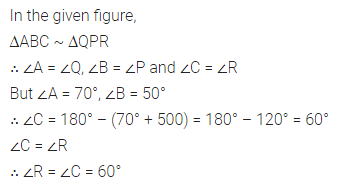Question 2.
In the given figure, ∆ABC ~ ∆QPR. The value of x is
(a) 2.25 cm
(b) 4 cm
(c) 4.5 cm
(d) 5.2 cmSolution:Question 3.
In the given figure, two line segments AC and BD intersect each other at the point P such that PA = 6 cm, PB = 3 cm, PC = 2.5 cm, PD = 5 cm, ∠APB = 50° and ∠CDP = 30°. Then, ∠PBA is equal to
(a) 50°
(b) 30°
(c) 60°
(d) 100°Solution:Question 4.
If in two triangles ABC and PQR,
$$\frac { AB }{ QR } =\frac { BC }{ PR } =\frac { CA }{ PQ }$$
then
(a) ∆PQR ~ ∆CAB
(b) ∆PQR ~ ∆ABC
(c) ∆CBA ~ ∆PQR
(d) ∆BCA ~ ∆PQR
Solution:Question 5.
In triangles ABC and DEF, ∠B = ∠E, ∠F = ∠C and AB = 3DE, then the two triangles are
(a) congruent but not similar
(b) similar but not congruent
(c) neither congruent nor similar
(d) congruent as well as similar
Solution:Question 6.
In the given figure, if D, E and F are midpoints of the sides BC, CA and AB respectively, then the two triangles ABC and DEF are
(a) similar
(b) congruent
(c) both similar and congruent
(d) neither similar nor congruentSolution:Question 7.
The given figure, AB || DE. The length of CD is
(a) 2.5 cm
(b) 2.7 cm
(c) $$\\ \frac { 10 }{ 3 }$$ cm
(d) 3.5 cmSolution: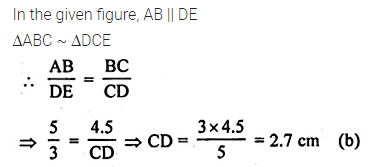Question 8.
If in triangles ABC and DEF,$$\frac { AB }{ DE } =\frac { BC }{ FD }$$ , then they will be similar when
(a) ∠B = ∠E
(b) ∠A = ∠D
(c) ∠B = ∠D
(d) ∠A = ∠F
Solution:Question 9.
If ∆PQR ~ ∆ABC, PQ = 6 cm, AB = 8 cm and perimeter of ∆ABC is 36 cm, then perimeter of ∆PQR is
(a) 20.25 cm
(b) 27 cm
(c) 48 cm
(d) 64 cm
Solution:Question 10.
In the given figure, DE || BC and all measurements are in centimetres. The length of AE is
(a) 2 cm
(b) 2.25 cm
(c) 3.5 cm
(d) 4 cmSolution: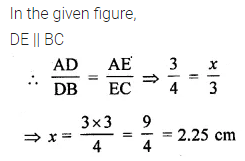Question 11.
In the given figure, PQ || CA and all lengths are given in centimetres. The length of BC is
(a) 6.4 cm
(b) 7.5 cm
(c) 8 cm
(d) 9 cmSolution:Question 12.
In the given figure, MN || QR. If PN = 3.6 cm, NR = 2.4 cm and PQ = 5 cm, then PM is
(a) 4 cm
(b) 3.6 cm
(c) 2 cm
(d) 3 cm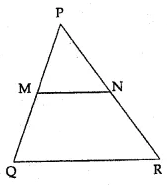Solution:Question 13.
D and E are respectively the points on the sides AB and AC of a ∆ABC such that AD = 2 cm, BD = 3 cm, BC = 7.5 cm and DE || BC. Then the length of DE is
(a) 2.5 cm
(b) 3 cm
(c) 5 cm
(d) 6 cm
Solution: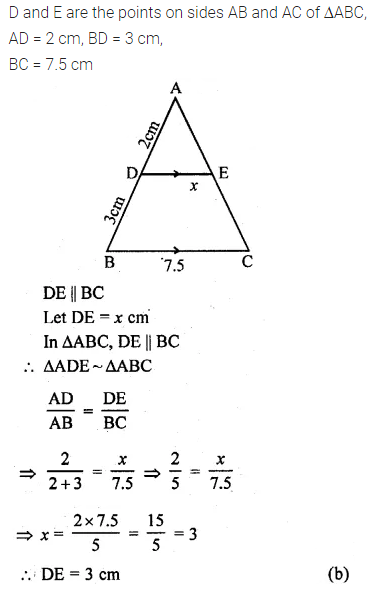Question 14.
It is given that ∆ABC ~ ∆PQR with $$\frac { BC }{ QR } =\frac { 1 }{ 3 }$$ then $$\frac { area\quad of\quad \Delta PQR }{ area\quad of\quad \Delta ABC }$$ equal to
(a) 9
(b) 3
(c) $$\\ \frac { 1 }{ 3 }$$
(d) $$\\ \frac { 1 }{ 9 }$$
Solution:Question 15.
If the areas of two similar triangles are in the ratio 4 : 9, then their corresponding sides are in the ratio
(a) 9 : 4
(b) 3 : 2
(c) 2 : 3
(d) 16 : 81
Solution:Question 16.
If ∆ABC ~ ∆PQR, BC = 8 cm and QR = 6 cm, then the ratio of the areas of ∆ABC and ∆PQR is
(a) 8 : 6
(b) 3 : 4
(c) 9 : 16
(d) 16 : 9
Solution:Question 17.
If ∆ABC ~ ∆QRP $$\frac { area\quad of\quad \Delta ABC }{ area\quad of\quad \Delta PQR }$$ AB = 18 cm and BC = 15 cm, then the length of PR is equal to
(a) 10 cm
(b) 12 cm
(c) $$\\ \frac { 20 }{ 3 }$$
(d) 8 cm
Solution:Question 18.
If ∆ABC ~ ∆PQR, area of ∆ABC = 81 cm², area of ∆PQR = 144 cm² and QR = 6 cm, then length of BC is
(a) 4 cm
(b) 4.5 cm
(c) 9 cm
(d) 12 cm
Solution: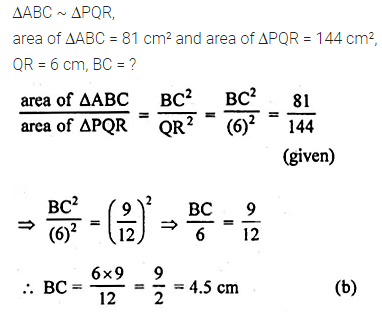Question 19.
In the given figure, DE || CA and D is a point on BD such that BD : DC = 2 : 1. The ratio of area of ∆ABC to area of ∆BDE is
(a) 4 : 1
(b) 9 : 1
(c) 9 : 4
(d) 3 : 2Solution:Question 20.
If ABC and BDE are two equilateral triangles such that D is mid-point of BC, then the ratio of the areas of triangles ABC and BDE is
(a) 2 : 1
(b) 1 : 2
(c) 1 : 4
(d) 4 : 1
Solution:Question 21.
The areas of two similar triangles are 81 cm² and 49 cm² respectively. If an altitude of the smaller triangle is 3.5 cm, then the corresponding altitude of the bigger triangle is
(a) 9 cm
(b) 7 cm
(c) 6 cm
(d) 4.5 cm
Solution:Question 22.
Given ∆ABC ~ ∆PQR, area of ∆ABC = 54 cm² and area of ∆PQR = 24 cm². If AD and PM are medians of ∆’s ABC and PQR respectively, and length of PM is 10 cm, then length of AD is
(a) $$\\ \frac { 49 }{ 3 }$$ cm
(b) $$\\ \frac { 20 }{ 3 }$$ cm
(c) 15 cm
(d) 22.5 cm
Solution: